# November 2018

Phase Transition of Finance

Chia Cheng Chang

Introduction to Two-phase Phenomena Observed in Finance

Phase transition in nature is understood to be emergent phenomena due to the collective behavior of individual particles. In the 􏰃nancial market, phase transitions are also observed while people replace the role of particles. In a demand-driven market, the collective behavior of buyers and sellers have lead Plerou, Gopikrishnan and Stanley to conclude that the collective tendency to buy or sell may be characterized as an order parameter. The tendency to buy or sell is quantitatively described by the volume imbalance, Ω(t), and undergoes a phase transition as the absolute deviation of Ω(t) exceeds a critical threshold Σc. In light of the observations made by Plerou, the model behind the two-phase phenomenon was explored by Zheng, Qiu and Ren. Zheng proposed that the two-phase phenomena could possibly be explained by either the minority games model or the herding model; the predictions made by the models were then compared to the German DAX in order to verify the validity of the theoretical models.

The demand of the market is quantfi􏰃ed by the volume imbalance functionΩ(t) which is de􏰃ned to be the difference between the number of buyer initiated transations QB and seller initiated transactions QS over a small time interval∆t. The equation below is taken from the article written by Plerou1.

N
Ω(t)≡QB −Qs =􏰋qiai (1)

i=1

Equation 1 describes the number of shares traded qi per transaction with N total transactions over a time interval ∆t. The values of ai = ±1 indicating repectively whether the transaction is buyer or seller initiated. Over any partic- ular interval, there will be a distribution on the number of shares traded; that is, if we analyze the distribution of the Ωi(t0) for any t0, it is possible to calculate the absolute deviation of Ω(t0). The absolute deviation of Ω(t) is de􏰃ned to be the 􏰀local noise intensity Σ􏰁1. Quantitatively the local noise intensity for a speci􏰃c time interval ∆t is de􏰃ned to be1

Σ(t) ≡ ⟨|qiai − ⟨qiai⟩|⟩ (2)

It was discovered that the probability distribution P(Ω,Σ) of the volume imbalance, and consequently the most probable value of Ω undergoes a phase transition as the local noise Σ exceeds a critical value Σc. Below the critical value, P(Ω,Σ) is a single peaked function centered around zero. This suggests that when buyers and sellers are generally inactive the mean value of the volume imbalance is zero and the market is in a stable equilibrium. However, the more interesting situation is when buyers and sellers become very active, possibly as

a result of market panic or news of impending doom on the future economy; Plerou observed that as the panic level reaches above the critical value Σc, the probability distribution P (Ω, Σ), becomes double peaked. As a result, players in the market will collectively end up either selling or buying in a situation where there is a large disparity in the number of shares traded; a situation most likely resulting from signs of depression or economic revival. The empirical evidence of such behavior is presented by Plerou and is reproduced below in Figure 1.

Figure 1: Three values of Σ are represented here. The solid black line indicates the region where Σ < Σc. The dotted red line plots the probability distribution when Σ = Σc. The dotted green line shows what happens when Σ > Σc. These graphs hold true for ∆t = 15mins up to half a day.1

From Figure 1, we see that for Σ below the critical value, the probability distribution of Ω, represented by the black line is sharply peaked at zero indi- cating the market being at a stable state. At the critical point, the distribution 􏰄attens out as shown by the red dotted line. For values of Σ greater than the critical value, the distribution undergoes a change and becomes double peaked. The empirical results discovered by Plerou suggest that socially, there is a pa- rameter in which the group behavior of participants in the market undergoes a phase transition. Understanding how this parameter may be measured in practice may provide an indication as to when the market will shift from a stable state to a dynamical state. Understanding qualitatively what P (Ω, Σ) indicates from emprical data prompted Potters and Bouchaud to discuss qualitatively the form of the probability distribution. The form of the probability distribution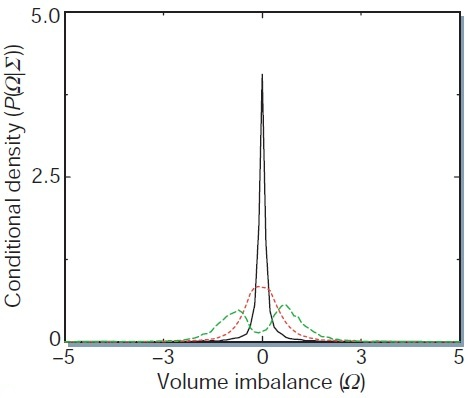function suggested by Potters is given to be2
1 􏰈 Ω2 􏰅βΩ2−Σ􏰆2􏰉

P(Ω,Σ)=Z(Σ)exp −2 − 2σ2 (3)

where Z(Σ) is the normalization while β and σ2 parameterize the magnitude and variance of the noise on Σ respectively. It is then natural to treat the term in the exponent as the action. After varying the action with respect to Ω and minimizing it, the classical solutions to the action are

1􏰌 σ2
Ωc=±√β Σ−2β (4)

As a result, we can conclude that the critical value for Σ is2σ2

Σc = 2β (5)

Given that only the real roots are kept, for values of Σ < Σc, the classical solution for Ω is zero. However, for values of Σ above the critical point, the classical solution yields two roots. Therefore, this hypothesis of the probability distribution function is in agreement with the empirical data gathered by Plerou. From equation 5, it can be seen that the value of Σc depend on the statistical characteristics of Σ. Therefore it was concluded by Potters that emergence of a two-phase phenomenon results from the nature of how trading is collectively executed. In the language of statistics, the critical value Σc is proportional to the variance while Σ itself describes the absolute deviation of Ω. This strongly suggests that the critical value Σc might be related to either the skewness or kurtosis of the volume imbalanace Ω. This was not a claim made by Potters, however, this appears to be an interesting direction for further investigation.

It is of interest to compare empirical evidence with current models used to analyze 􏰃nancial markets. While empirically the transition between a single peak to double peaked probability distribution function is due to the statistics of Σ, theoretically, emergent behavior is a result of a model exhibiting long range correlations. In the 􏰃nancial markets, these are long ranged temporal correlations; the participants in these models make decisions to buy or sell according to results which occured in past time steps. Zheng, Qiu and Ren investigated the possibility of reproducing the emergent behavior though the minority games model.3The Minority Games

The minority game is a generic model used to describe competing and adap- tive participants in the economy.4 In this game, there are N agents who are forced to select one of two choices and the reward is awarded to the minority choice. In order to make this choice, each agent is given a set number of S strate- gies which they will use to determine the choice they will pick. To more closely model a real economy, agents may be divided into speculators and producers. Speculators are characterized as being more versatile in their strategies and therefore are given more strategies to determine their choice. Producers how- ever, are less 􏰄exible, possibly due to 􏰃xed assets associated with the industry and therefore are given less strategies. In Zheng’s approach, a modi􏰃ed version of the original minority game was applied and an inactive state was added in order to allow the size of the participants to vary. This is the manifestation of long range temporal correlation in this model.3 The resulting probability distri- bution function which arises from the minority games model is shown in 􏰃gure 2.3

Figure 2: The axis here is equivalent to Figure 1. Z ≡ Ω and r ≡ Σ. There are 501 speculators and 1000 producers. Here speculators have an inactive strategy while producers are forced to play. Both speculators and producers are given s=2 strategies and base their decision on the outcome of the strategies on up to m=2 time steps before the current state. Probability distributions for di􏰂erent noise levels (r) are plotted.

From 􏰃gure 2 it can be seen that for increasing values of Σ (denoted by r in the 􏰃gure), the probability distribution gains two peaks. However, the peak

4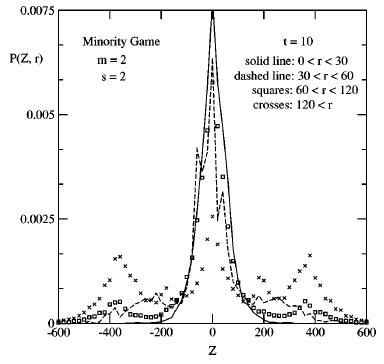centered around zero does not disappear as values of Σ increase. This is in dis- agreement with the empirical data described by 􏰃gure 1. This result suggests that the minority games model is inadequate in describing the two-phase phe- nomena since the probability distribution of volume imbalance simulated does not evolve in the same way that was observed experimentally. Zheng suggests that the origin of the descrepency may be traced back to the periodic nature of the minority games. The periodic nature of the solution is a result of the par- ticipants having short term memory.6 In the simulation that Zheng proposed, the participants were set to make decisions based on experiences up to m=2 time steps in the past. In the introductory guide to minority games written by Moro, he points out that if only recent information is used to make decisions, then the participants will periodically play the game in exactly the same way.

Figure 3: Time evolution of Attendence for m=2, 7, 15 from top to bottom. Periodic pattern observed for m=2 and 7.6 Attendence is de􏰃ned to be the sum of all options A(t) = 􏰊ai for ai = ±1. Here the two options are given a numerical value ±1 although the contents of the options may be arbritrary (eg. go to Disneyland or stay home and watch TV).

Figure 3 is a graph from Moro’s paper showing that for relatively lower values of m, the way the game is played out, quanti􏰃ed by the function A(t), will be periodic in nature. This is especially clear for the m=2 case which Zheng’s simulation was set to. One possible avenue for further investigation of the minority games model is to increase the parameter m to a large enough value (for example m=15 as suggested by Moro). If indeed the origin of the disagreement between theory and experiment stems from the periodic nature of the minority games, then allowing participants with bigger brains and longer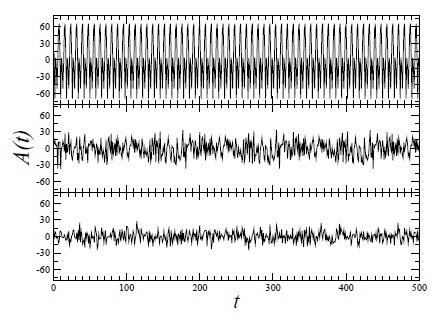memory retention rates may be the next step towards improving this model.

The Herding Model

The herding model describes a system of interacting agents who share in- formation and make decisions based on the collective action of the group. The model was 􏰃rst introduced by Eguiluz and Zimmermann, hence is also called the EZ herding model. In the paper Eguiluz published5, the algorithm of the herding model is described as follows. The herd is played by N agents. The state of agent i is described by φi = {−1,0,1} corresponding to selling, inac- tive or buying state respectively. Initially all agents are inactive, however for each time step, one agent (denoted by agent j) selected at random is allowed to become active (φ = ±1) with probability described by a constant a. Interac- tion between the agents is introduced by allowing agents to share information. This is described by having inactive agents form links with other agents. When an agent belonging in the cluster becomes active, the cluster then immediately make the same decision as the active agent. After the decision to act is made my the cluster, all links within the cluster are then removed. After the decision to buy or sell is made, all agents in the cluster are again set to the inactive state, however, agent j (the initiator) has only a probability of (1 − a) of becoming inactive. If agent j becomes inactive, then the agent will establish a link with other agents and become part of a cluster. The whole process is then repeated. If agent j stays active however, it will only be a singular decision as agent j in this case is not part of any cluster.

Applied to the real market, the N agents describe participants in the market. At any given time step, most participants are inactive because there are no rumors or information to indicate whether buying or selling is bene􏰃cial. During inactivity however, Eguiluz suggests that participants may use similar analysis tools and arrive at similar opinions on the current market situation. Therefore, when a participant belonging in the group is motivated to act due to a rumor or other relevent information (insider trading?), other members will immediately come to the same conclusion and act in the same manner. The reason why all link are dropped after the cluster becomes active is because any information and opinion pertaining to that particular market situation will no longer be relevent anymore.5 Finally, the initiator, agent j, is given a probability (1 − a)of becoming inactive probably because the person is in a position that allows him to make more informed decisions, possibly legally. A more logical reason though, is to ensure that as the parameter a is set to 1, that the model approaches a logical steady state solution.

The most important parameter in this model is the probability of activation a. Therefore to check that this model behaves as expected, extremum values for the parameter a should be discussed. If the parameter a is set to 1, then any time an initiator is chosen, agent j will become active. Also, due to the fact that agent j possesses a (1 − a) probability of becoming inactive, with a = 1, the agent will never become inactive. The equilibrium state of this market will result

in all agents acting individually with no group behavior. In the other extreme, by setting a ≪ 1 participants will have an increasing tendency of forming large clusters since agent j is almost never active to trigger o􏰂 an event which then breaks the links. Therefore for small values of a, the model describes highly correlated group behavior. It is then suitable to de􏰃ne a parameter5

h ≡ ‘1 − 1 (6)a

de􏰃ned to be the herding parameter. For small values of a, we observe large group behavior and therefore is associated with a large herding param- eter. When a = 1, no group behavior is observed and therefore we arrive at a herding parameter of zero. In real life, the herding parameter describes the rate of information dispersion.5 The faster information is passed around, the more likely larger and larger groups of people will act according to the same information while on the other extreme, if nobody talks then everyone may only act individually.

From 􏰃gure 2, we see that the minority games model did not adequately capture the two-phase phenomena empirically observed by Plerou as shown in 􏰃gure 1. Therefore, as a separate attempt, Zheng tried the EZ herding model. The distribution for P (Ω, Σ) resulting from the simulation as well as the empirical data of the German DAX is shown in 􏰃gure 4 for comparison.3

Figure 4: EZ model: Probability distribution P (Ω, Σ) for N=10,000 agents, h=19. DAX(94-97): Empirical data of P (Ω, Σ). Note that the time of t=100 in the EZ model scales to 10 minutes in real time as is used to generate the DAX plot.3 Notation used: Ω ≡ Z and Σ ≡ r.

Figure 4 shows that the prediction of the herding model results in the double peaked probability distribution with increasing values of Σ. This is in agreement with the empirical data described by the German DAX presented also in 􏰃gure 4. Qualitatively however, the shape of the peaks do not agree with the empirical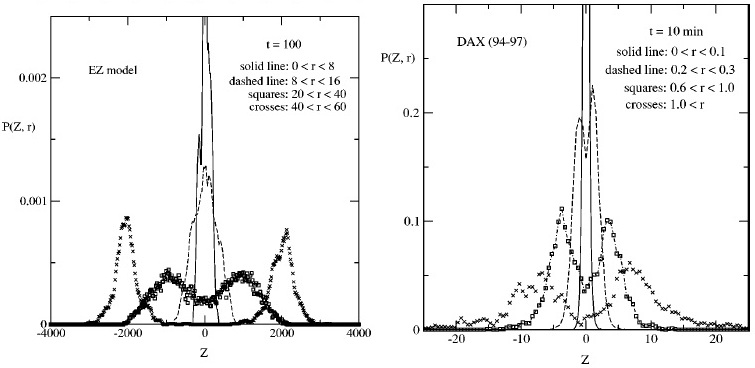data. More speci􏰃cally, for increasing values of Σ, empirically the height of the peaks decrease while the width broadens out as shown in the DAX plot. The EZ model however, shows that the peaks sharpen and increase in height as seen when comparing the probability distribution represented by the squares and crosses. Zheng suggests that the reason why the scaling with respect toΣ of the standard EZ herding model is in disagreement with empirical data is because the herding model only allows for short ranged temporal correlations while in real market situations the correlations are long ranged. The standard herding model is short ranged due to the fact that the herding parameter is held to be a constant throughout the simulation. This however, is not true in real market situations since the rate of information transmission should vary depending on the current market situation.3 A way to incorporate varying rates of transmission is introduced by Zheng in the interacting herding model.

Interacting EZ Herding Model

In the interacting EZ herding model, Zheng suggests that when the market is very sensitive (when no one knows what is going on and therefore characterized by small cluster sizes), agents and news agencies are attentive and prompt at responding to and reporting news regarding the status of the market. However, when the market is stable and there is less interest in the market, the opposite happens; people will form large clusters. Using this idea, Zheng proposed that the probability of activating agent j should equal3

a[s]|t=t′ = b + cs−δ 􏰇􏰇t=t′ −1 (7)

where s(t) is the average size of a cluster at time t, while b, c and δ are constants. An e􏰂ect of de􏰃ning the probability of activation with respect to the cluster size of the previous time step is also pertinent. If a large cluster was activated at t′ − 1 then the average cluster size s would drop rapidly due to the links being dropped. As a result the herding parameter will become big and will allow for larger clusters to form at t′. If a smaller cluster was activated at t′ −1then the average cluster size would have a relatively smaller decrease and the herding parameter will only increase by a small amount. In real situations this would possibly suggest that large clusters (analysis who use the same program or receive the same information) will continue to remain active with a high herding parameter even after the cluster dissolves; on the other hand folks who buy or sell for idiosyncratic reasons (eg. when they see a shooting star) tend to be the only ones who act that way and do not usually gain a large following of investors willing to imitate. Therefore for activation of small clusters, the impact on the herding parameter is very small. With the addition of what amounts to long ranged temporal correlations, the interacting herding model is used to simulate the probability distribution of volume imbalance again and the results are shown in 􏰃gure 5.3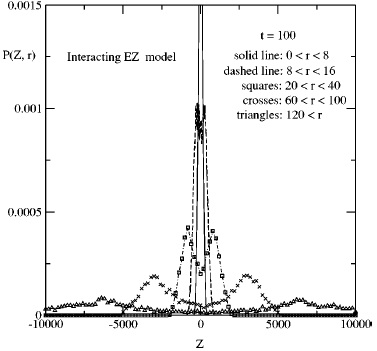Figure 5: Interacting EZ herding model for N=10,000 agents, b=0.001, c=0.6 andδ=1. Notation: Ω≡Z andΣ≡r.

The problem with the standard EZ herding model is that for increasing val- ues of Σ, the peaks of P(Ω,Σ) do not scale down properly. For the interacting herding model, Zheng claims that the problem has been solved. Refering to 􏰃gure 5, we see that compared to the standard herding model, for values in increasing Σ, the peaks 􏰄atten out and decrease in magnitude as suggested by empirical data. The phase transition from a single peaked probability distribu- tion to a double peaked distribution is also observed. Therefore qualitatively the interacting EZ herding model contains all the important phenomena associ- ated with the two-phase behavior observed empirically by both Plerou and the German DAX. In 􏰃gure 6, Zheng compares the DAX data with the interacting EZ herding model directly. Although the plots have been rescaled by a constant factor, the DAX data 􏰃ts perfectly with the interactive EZ model. Therefore, allowing the herding parameter to vary as a function of cluster size was vital in having the herding model behave similarly to the observed phenomena. The result is very exciting because it would suggest that there is qualitatively a ro- bust model to describe the observed phase transition in 􏰃nance. The next step would be to understand the connection between experimental and theoretical values of Ω, Σ and t in order to make quantitative comparisons between the two sets of data.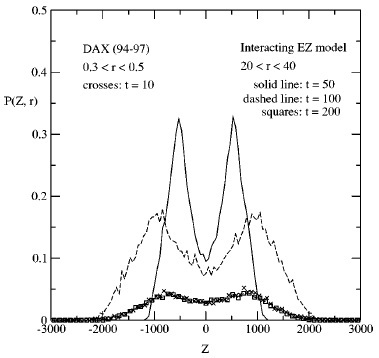Figure 6: Interacting EZ herding model for N=10,000 agents, b=0.001, c=0.6,δ = 1. DAX o􏰂ers experimental data for comparison. Note3: The plots have been rescaled by constant factors. Notation: Ω ≡ Z and Σ ≡ r.

Conclusion

The observation of two-phase phenomena in 􏰃nance sparked great interest particularly in the physics community to understand this socio-economic behavior through classical phase transition methods. In a theoretical standpoint, the probability distribution function suggested by Potters as shown in equation 3 allows for a path integral type formalism understanding of the phenomena; while the transition from a single-valued peak to a double-valued peak is reminiscent to spontaneous symmetry breaking. Further investigation from the direction of statistics is also possible as the kurtosis of the distribution describes the unique shape of the tails and is related to the critical point of the phase transition Σc. The minority games allow for a possible route of understanding these phenomena through a very simpli􏰃ed model of 􏰃nancial markets. Although the model was unsuccessful in describing the phenomena, the possibility of removing the periodic nature of the minority games is worthy for further investigation. The herding model and ultimately the interacting herding model qualitatively describes the phenomena observed initially my Plerou. The success of the interacting herding model is very interesting because it suggests that humans really do tend to act like sheep during decision making. This may be a concept understood ever since grade school, however, to be able to simulate the same behavior with very simple but important considerations (such as varying the herding parameter with cluster size) is incredibly fascinating. As a practical application,

10

understand which phase the market is in and when the market will switch phases may also be important for 􏰃nancial risk management. In the single peak phase, odds are no matter how reckless one is on buying stock, there is considerably little risk since there is no net demand to sell or buy and prices as a result, will be relatively stable. In the double-peaked phase, there is considerably more risk associated as the market is in search of a new equilibrium price. In any case, the 􏰃eld of quantitative 􏰃nance and behavior 􏰃nance is relatively new; especially true for new 􏰃elds is the possibility to be the 􏰃rst in discovering something new and impactful. The chance of modeling human behavior in a scienti􏰃c way (as opposed to fortune telling) is no doubt very exciting.

References
 V. Plerou, P. Gopikrishnan, and H.E. Stanley, Nature 421, 130 (2003).  M. Potters, and J.-P. Bouchaud, arXiv:cond-mat/0304514v1 (2003).
 B. Zheng, T. Qiu, and F. Ren, Phys. Rev. E 69, 046115 (2004).
 D. Challet, M. Marsili, and Y.-C. Zhang, Physica A 299, 228-233 (2001).  V. Eguiluz, and M. G. Zimmermann, Phys. Rev. Lett. 85, 5659 (2000).  E. Moro, arXiv:cond-mat/0402651v1 (2004)

11